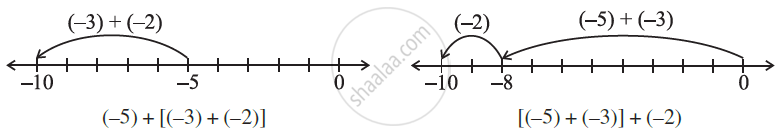# Properties of Addition and Subtraction of Integers:

We have learned that sum of two whole numbers is again a whole number. For example, 17 + 24 = 41 which is again a whole number. We know that this property is known as the closure property for the addition of the whole numbers.

### Observation

17 + 23 = 40 Result is an integer
(- 75) + 18 = - 57 Result is an integer
27 + (- 27) = 0 Result is an integer
( - 20) + 0 = - 20 Result is an integer
(– 35) + (– 10) = - 45 Result is an integer

Since the addition of integers gives integers, we say integers are closed under addition.
In general, for any two integers a and b, a + b is an integer.

## 2. Closure under Subtraction:

We have learned that the subtraction of two whole numbers is again a whole number. For example, 17 - 24 = - 7 which is again a whole number. We know that this property is known as the closure property for the subtraction of the whole numbers.

### Observation

7 - 9 = - 2 Result is an integer
( -21) - ( -10) = - 11 Result is an integer
32 - ( -17) = 49 Result is an integer
( -18) - (-18) = 0 Result is an integer
(- 29) - 0 = - 29 Result is an integer

As you can see that the subtraction of two integers will always be an integer, hence integers are closed under subtraction
Thus, if a and b are two integers then a – b is also an integer.

## 3. Commutative Property:

We know that 3 + 5 = 5 + 3 = 8, that is, the whole numbers can be added in any order. In other words, addition is commutative for whole numbers.
Similarly,

(i) (– 8) + (– 9) and (– 9) + (– 8) = - 17
So, (– 8) + (– 9) = (– 9) + (– 8).

(ii) (– 23) + 32 and 32 + (– 23) = 9
So, (– 23) + 32 = 32 + (– 23).

(iii) (- 47) + 0 and 0 + ( - 47) = - 47
So, (- 47) + 0 = 0 + ( - 47).

Thus, Integers can be added in any order.
In other words, addition is commutative for integers i.e., a + b = b + a for all integers a, and b.

• Consider the integers 5 and (– 3).
Is 5 – (– 3) the same as (– 3) – 5?
No, because 5 – (– 3) = 5 + 3 = 8, and (– 3) – 5= – 3 – 5 = – 8.
We conclude that subtraction is not commutative for integers.

## 4. Associative Property:

Consider the integers – 3, – 2, and – 5.

Look at (– 5) + [(– 3) + (– 2)] and [(– 5) + (– 3)] + (– 2).

In the first sum (– 3) and (– 2) are grouped together and in the second (– 5) and (– 3) are grouped together. We will check whether we get different results.In both cases, we get – 10. i.e., (– 5) + [(– 3) + (– 2)] = [(– 5) + (– 2)] + (– 3)

Addition is associative for integers, i.e., (a + b) + c = a + (b + c) for all integers a, b and c.

When we add zero to any whole number, we get the same whole number. Zero is an additive identity for whole numbers. For example, 0 + 59 = 59, 0 + 37 = 37.

Similarly, When we add zero to any integer number, we get the same integer number. Zero is an additive identity for an integer number.

### Observation

( - 23) + 0 = - 23 Result is an integer
- 61 + 0 = - 61 Result is an integer
56 + 0 = 56 Result is an integer
0 + 0 = 0 Result is an integer

Integer 0 is the identity under addition. That is, a + 0 = 0 + a = a for every integer a.

If you would like to contribute notes or other learning material, please submit them using the button below.

### Shaalaa.com

Properties Of Addition & Subtraction Of Integers [00:17:06]
S
0%